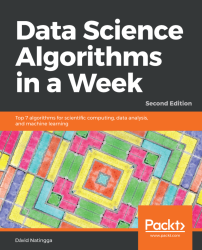•#### Data Science Algorithms in a Week - Second Edition#### Overview of this book

Machine learning applications are highly automated and self-modifying, and continue to improve over time with minimal human intervention, as they learn from the trained data. To address the complex nature of various real-world data problems, specialized machine learning algorithms have been developed. Through algorithmic and statistical analysis, these models can be leveraged to gain new knowledge from existing data as well. Data Science Algorithms in a Week addresses all problems related to accurate and efficient data classification and prediction. Over the course of seven days, you will be introduced to seven algorithms, along with exercises that will help you understand different aspects of machine learning. You will see how to pre-cluster your data to optimize and classify it for large datasets. This book also guides you in predicting data based on existing trends in your dataset. This book covers algorithms such as k-nearest neighbors, Naive Bayes, decision trees, random forest, k-means, regression, and time-series analysis. By the end of this book, you will understand how to choose machine learning algorithms for clustering, classification, and regression and know which is best suited for your problem
Title PagePackt UpsellContributorsPrefaceFree Chapter
Classification Using K-Nearest NeighborsNaive BayesDecision TreesRandom ForestsClustering into K ClustersRegressionTime Series AnalysisPython ReferenceStatisticsGlossary of Algorithms and Methods in Data ScienceOther Books You May EnjoyIndex## Weight prediction from height – linear regression on real-world data

Here, we are predicting the weight of a man from his height by using linear regression on the following data:

 Height in cm Weight in kg 180 75 174 71 184 83 168 63 178 70 172 ?

We would like to estimate the weight of a man given that his height is 172 cm.

### Analysis

In the previous example of Fahrenheit and Celsius conversion, the data fitted the linear model perfectly. Thus, we could perform even a simple mathematical analysis (solving basic equations) to gain the conversion formula. Most data in the real world does not fit a model perfectly. For such an analysis, it would be good to find a model that fits the given data with minimal errors. We can use the least squares method to find such a linear model.

Input:

We put the data from the preceding table into the vectors and try to fit the linear model:

```# source_code/6/weight_prediction.py
import numpy as np
from scipy.linalg import lstsq

height = np.array([180,174,184,168,178])
weight = np...```